Loading...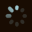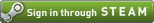OR  Zero-K Name:    Password:

# WHR Teams, FFA and Variance

3 posts, 239 views
Filter:    Player:
sort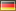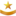Brackman
9 months ago
I'd have some questions on how WHR is currently implemented that are not answered in the WHR paper.

1. Are teams rated by averaging their WHR first and applying the exponential function afterwards or the other way round?

2. Are FFAs predicted by probabilities proportional to the teams' exp(WHR) or proportional to an average of a team's win probabilities against all other teams? The latter would also make sense according to an earlier calculation.

3. Is the visible WHR deviation currently measured by variance or standard deviation?

4. Is the rating variability w = sqrt(14/day) Elo or a different number?

From the current results of WHR, I guess the answer is (1st, 2nd, 1st, ?) and should be (1st, 2nd, 2nd, ?).
+0 / -0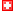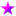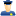DeinFreund
9 months ago
 quote:1. Are teams rated by averaging their WHR first and applying the exponential function afterwards or the other way round?
The Elo rating is averaged. Then the exponential function is applied.

 quote:2. Are FFAs predicted by probabilities proportional to the teams' exp(WHR) or proportional to an average of a team's win probabilities against all other teams? The latter would also make sense according to an earlier calculation.
FFA is first reduced to one number per allyteam by averaging all Elos. Then it is treated as a series of 1 vs everyone, where all enemy teams are averaged to get an opponent Elo. The win chances are then normalized.

I haven't experimented much with other approaches here, calculating the exponential directly after averaging the teams might be a good solution too.

 quote:3. Is the visible WHR deviation currently measured by variance or standard deviation?

Variance.

 quote:4. Is the rating variability w = sqrt(14/day) Elo or a different number?

The elo range expands as sqrt(35 * days). This assumes that every player has an expected random change of +-35 Elo each day.
+0 / -0Brackman
Thx for the answers.

The answer to 1. seems to be ideal.

And by series of 1 vs everyone you mean the sum over all other teams of 1 vs other team? If yes, 2. is what I would think is ideal, too.

For visible deviation, I think the standard deviation should be used instead of variance, the square root of the covariance matrix' diagonal elements. The expected deviation of a players skill is assumed to increase as in a random walk, proportional to sqrt(time). But if variance is used, it is proportional to time.

sqrt(35*time/day) seems a bit much. Did you test that this yields better results than the standard sqrt(14*time/day)?
+1 / -0